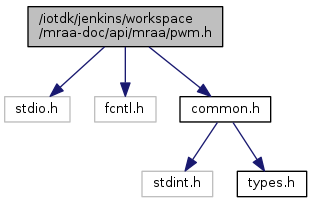mraa  2.0.0 Low Level Skeleton Library for Communication on GNU/Linux platforms
pwm.h File Reference

## API Description

PWM is the Pulse Width Modulation interface to libmraa. It allows the generation of a signal on a pin. Some boards may have higher or lower levels of resolution so make sure you check the board & pin you are using before hand.

Go to the source code of this file.

## Functions

mraa_pwm_context mraa_pwm_init (int pin)

mraa_pwm_context mraa_pwm_init_raw (int chipid, int pin)

mraa_result_t mraa_pwm_write (mraa_pwm_context dev, float percentage)

mraa_result_t mraa_pwm_period (mraa_pwm_context dev, float seconds)

mraa_result_t mraa_pwm_period_ms (mraa_pwm_context dev, int ms)

mraa_result_t mraa_pwm_period_us (mraa_pwm_context dev, int us)

mraa_result_t mraa_pwm_pulsewidth (mraa_pwm_context dev, float seconds)

mraa_result_t mraa_pwm_pulsewidth_ms (mraa_pwm_context dev, int ms)

mraa_result_t mraa_pwm_pulsewidth_us (mraa_pwm_context dev, int us)

mraa_result_t mraa_pwm_enable (mraa_pwm_context dev, int enable)

mraa_result_t mraa_pwm_owner (mraa_pwm_context dev, mraa_boolean_t owner)

mraa_result_t mraa_pwm_close (mraa_pwm_context dev)

int mraa_pwm_get_max_period (mraa_pwm_context dev)

int mraa_pwm_get_min_period (mraa_pwm_context dev)

## Typedefs

typedef struct _pwm * mraa_pwm_context

## Function Documentation

 mraa_result_t mraa_pwm_close ( mraa_pwm_context dev )

Close and unexport the PWM pin

Parameters
 dev The pwm context to use
Returns
Result of operation
 mraa_result_t mraa_pwm_enable ( mraa_pwm_context dev, int enable )

Set the enable status of the PWM pin. None zero will assume on with output being driven. and 0 will disable the output.

Parameters
 dev The pwm context to use enable Toggle status of pin
Returns
Result of operation.
 int mraa_pwm_get_max_period ( mraa_pwm_context dev )

Get the maximum pwm period in us

Parameters
 dev The pwm context to use
Returns
max pwm in us
 int mraa_pwm_get_min_period ( mraa_pwm_context dev )

Get the minimum pwm period in us

Parameters
 dev The pwm context to use
Returns
min pwm in us
 mraa_pwm_context mraa_pwm_init ( int pin )

Initialise pwm_context, uses board mapping

Parameters
 pin The PWM PIN
Returns
pwm context or NULL
 mraa_pwm_context mraa_pwm_init_raw ( int chipid, int pin )

Initialise pwm_context, raw mode

Parameters
 chipid The chip inwhich the PWM is under in SYSFS pin The PWM PIN.
Returns
pwm context or NULL
 mraa_result_t mraa_pwm_owner ( mraa_pwm_context dev, mraa_boolean_t owner )

Change ownership of context

Parameters
 dev the context owner Ownership boolean
Returns
Result of operation
 mraa_result_t mraa_pwm_period ( mraa_pwm_context dev, float seconds )

Set the PWM period as seconds represented in a float

Parameters
 dev The Pwm context to use seconds Period represented as a float in seconds
Returns
Result of operation
 mraa_result_t mraa_pwm_period_ms ( mraa_pwm_context dev, int ms )

Set period, milliseconds.

Parameters
 dev The Pwm context to use ms Milliseconds for period
Returns
Result of operation
 mraa_result_t mraa_pwm_period_us ( mraa_pwm_context dev, int us )

Set period, microseconds

Parameters
 dev The Pwm context to use us Microseconds as period
Returns
Result of operation
 mraa_result_t mraa_pwm_pulsewidth ( mraa_pwm_context dev, float seconds )

Set pulsewidth, As represnted by seconds in a (float)

Parameters
 dev The Pwm context to use seconds The duration of a pulse
Returns
Result of operation
 mraa_result_t mraa_pwm_pulsewidth_ms ( mraa_pwm_context dev, int ms )

Set pulsewidth, milliseconds

Parameters
 dev The Pwm context to use ms Milliseconds for pulsewidth
Returns
Result of operation
 mraa_result_t mraa_pwm_pulsewidth_us ( mraa_pwm_context dev, int us )

Set pulsewidth, microseconds

Parameters
 dev The Pwm context to use us Microseconds for pulsewidth
Returns
Result of operation
 float mraa_pwm_read ( mraa_pwm_context dev )

Read the output duty-cycle percentage, as a float

Parameters
 dev The Pwm context to use
Returns
percentage A floating-point value representing percentage of output. The value should lie between 0.0f (representing on 0%) and 1.0f Values above or below this range will be set at either 0.0f or 1.0f
 mraa_result_t mraa_pwm_write ( mraa_pwm_context dev, float percentage )

Set the output duty-cycle percentage, as a float

Parameters
 dev The Pwm context to use percentage A floating-point value representing percentage of output. The value should lie between 0.0f (representing on 0%) and 1.0f Values above or below this range will be set at either 0.0f or 1.0f
Returns
Result of operation

## Typedef Documentation

 typedef struct _pwm* mraa_pwm_context

Mraa Pwm Context

Include dependency graph for pwm.h: